# How Computers Really Work: Math via Boolean Logic

As I try to write more posts about ARM programming, I’m finding that I keep getting sidetracked by background stuff. Hopefully it’s interesting; if not, let me know in the comments!

Today’s sidetrack: how the heck does a computer actually do math?

As I said in my post about binary the other day, at a deep level, computers don’t actually work with numbers, not even zeros and ones. Computers are electronic devices, and what they really do is worth with electrical signals. In computer hardware, there are really just a few fundamental operations that it can perform with those signals. By taking those basic operations, and combining them in the right way, we can do math. How that works is very mysterious to most people. Obviously, I can’t describe all of how a computer works in a blog post. But what I’m going to do is explain the basic parts (the gates that are used to build the hardware), and how to combine them to implement a piece of hardware that does integer addition.

In computer hardware, there are four fundamental components that we can use to build operations, and they’re the basic operations of boolean logic: And, Or, Exclusive Or, and Not.

In talking about these kinds of operations, we frequently use truth tables to explain them. A truth table just lists all of the possible input combinations, and tells you what the output for them is. For example, here’s a truth table showing you AND, OR, and XOR:

A B A∧B A∨B A⊕B
0 0 0 0 0
0 1 0 1 1
1 0 0 1 1
1 1 1 1 0

In terms of hardware, you can actually implement all of these using one primitive gate type, the NAND (negated AND) gate. For example, in the diagram below, you can see how to build a NOT gate using a NAND gate, and then using using that NAND-based NOT gate, to build an AND gate using two NANDs.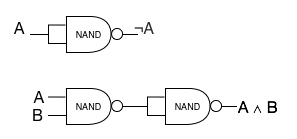In the hardware, those gates are all that we have to work with. To build arithmetic, we need to figure out how to combine these primitive pieces to build up something that produces the right results. First, we need to be able to describe what the correct results are. We’ll start by figuring out what it means to add two 1-bit numbers. We’ll have two one-bit inputs. We need two outputs – because two one-bit numbers can add up to a two-bit sum. We’ll call the outputs S and C (sum and carry – S is the low-order bit, which would be the sum if we could only have a one-bit result, and C is the value of the second bit.)

We can describe addition with a truth table:

A B S C
0 0 0 0
0 1 1 0
1 0 1 0
1 1 0 1

We can read those rows as “If A is 0 and B is 0, then A+B is 0 with a carry of 0”.

If we compare that table to the table up above showing the basic operations, then we can see that S is exactly the same as XOR, and C is exactly the same as AND. So we can build a one-bit adder out of gates: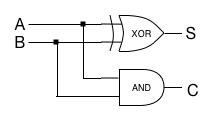This little one-bit adder is commonly called a half-adder, because to really implement a useful add operation, we need two of them for each bit.

If you think about what you’d need to do to add together two two-bit numbers, you couldn’t just use a half-adder for each bit. The carry from the first bit needs to get added to the second bit. You need to plumb the carry result from the first bit into a third input for the second bit. To get that second bit to work properly, you need to add together three one-bit values: the two inputs for the high-order bit, and the carry from the low-order bit. Generalizing, if you want to add together N bits,
when you’re computing the sum of the Mth bit, you need to include the carry from the M-1th bit.

To include the carry, we need to combine half-adders into a full adder, which looks like the following: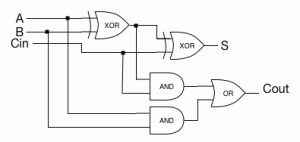Using that, we can create an N-bit adder, by chaining the carry output from bit M-1 to the carry input of bit M. This creates the simplest adder circuit, which is called a ripple-carry adder.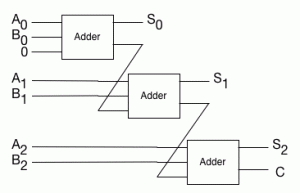Ripple-carry adders are the simplest way of building an integer addition operation in hardware. They’ve got one very big downside: to add the Nth bit, you need to have the result of adding the N-1th bit. That means that the more bits you add, the slower the ripple-carry adder gets. You have to way for the signals to propagate all the way through the circuit from the low bits to the high bits.

So ripple-carry isn’t really used in hardware anymore. There are a bunch of more complicated ways of building the adder that get rid of that propagation delay. It comes down to a very common tradeoff for engineers: performance versus complexity. But at the end of the day, the concept is still basically the same: it’s still a chain of multiple simple adders, that work the way I described here.

# Representing Numbers in Binary

Before we can really start writing interesting programs with our ARM, even simple ones, we need to understand a bit about how how a computer actually works with numbers.

When people talk about computers, they frequently say something like “It’s all zeros and ones”. That’s wrong, in a couple of different ways.

First, when you look at the actual computer hardware, there are no zeros and ones. There are two kinds of signals, represented as two different voltage levels. You can call them “high” and “low”, or “A” and “B”, or “+” and “-“. It doesn’t matter: it’s just two signals. (In fact, one fascinating thing to realize is that there’s a fundamental symmetry in those signals: you can swap the choice of which signal is 0 and 1, and if you just swap the and gates and the or gates, everything you won’t be able to tell the difference! So one ARM processor could use one signal for 1, and a different ARM could use that signal as 0, and you wouldn’t actually be able to tell. In fact, everyone does it the same way, because chip design and manufacture are standardized. But mathematically, it’s possible. We’ll talk about that duality/symmetry in another post.)

Second, the computer really doesn’t work with numbers at all. Computer hardware is all about binary logic. Even if you abstract from different voltages to the basic pairs of values, the computer still doesn’t understand numbers. You’ve got bits, but to get from bits to numbers, you need to decide on a meaning for the bits two possible values, and you need to decide how to put them together.

That’s what we’re really going to focus on in this post. Once you’ve decided to call on of the two primitive values 1, and the other one 0, you need to decide how to combine multiple zeros and ones to make a number.

It might seem silly, because isn’t it obvious that it should use binary? But the choice isn’t so clear. There have been a lot of different numeric representations. For one example, many of IBM’s mainframe computers used (and continue to use) something called binary coded decimal (BCD). It’s not just different for the sake of being different: in fact, for financial applications, BCD really does have some major advantages! Even if you have decided to use simple binary, it’s not that simple. Positive integers are easy. But how do you handle negative numbers? How do you handle things that aren’t integers?

We’re going to start with the simplest case: unsigned integers. (We’ll worry about fractions, decimals, and floating point in another post.) Like pretty much all modern computers, the ARM uses the basic, mathematical binary exponential representation. This works basically the same as our usual base-10 numbers. We look at the digits from right to left. The leftmost digit (also called the least significant digit counts ones; the next digit counts 10s; the next counts 100s, and soon. So in base 10, the number 3256 means 6*100 plus 5*101 plus 2*102 plus 3*103.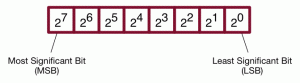In binary, we do exactly the same thing, only we do it with powers of 2 instead of powers of 10. So in binary the number 1001101 is 1 + 0*21 + 1*22 + 1*23 + 0*24 + 0*25 + 1*26 =1 + 4 + 8 + 64 = 77.

Arithmetic on binary is easy enough – you do the same thing you would with decimal, but in subtraction, borrows give you 2, not 10. As a quick example, let’s look at 7 + 13, which is 111 + 1101.

1. We start at the right edge. We have 1 + 1 = 10 – so the first digit of the sum is 0, and we carry 1.
2. Next we have 1 + 0 + 1(carry) = 10 – so the second digit is again 0, and we carry 1. Our sum so far is 00.
3. Now we’re on to the third digit. 1 + 1 + 1(carry) = 11. So the third digit is 1, and we carry one. Our sum so far is 100.
4. Now the fourth digit, 1 + 0 + 1(carry), so we get 10. So the sum is 10100, or 20.

We’ll ignore subtraction for a moment, because as we’ll see in a little while, in computer hardware, we don’t actually need to have subtraction as a primitive. Addition is the core of what computer arithmetic, and we’ll use addition to implement subtraction by taking the negative of the number being subtracted. (That is, to compute A-B, we’ll do A+(-B).)

Positive integers with addition aren’t enough to do most stuff we want to do on a computer. Even if we’re never going to write a program that manipulates numbers, we absolutely need to be able to subtract. To a computer, the only way to compare values is to subtract one from another! So we need to be able to do negatives and subtractions. How can we represent negative numbers in binary?

There are three basic choices, called sign-bit/sign-magnitude, one’s-complement and two’s complement. I’ve put an example of the three representing the number 75 in the figure below.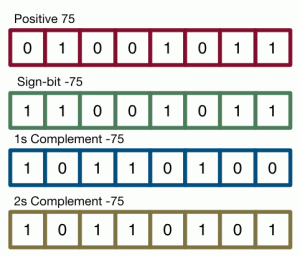In sign-bit representation, what you do is take the leftmost bit (also called the high-order bit), and use it to indicate sign (for obvious reasons, you call it the sign bit.). If the sign bit is 0, then the number is positive; if it’s 1, then the number is negative. For example, 01010 would be +10; 11010 would be -10.

For a human being, sign-bit looks great. But in practice, sign-bit was never used much, because while it looks simple to a human, it’s quite complicated to build in computer hardware. IBM did use it in some early machines, but even they gave up on it.

Next is one’s complement. In 1’s complement, high order bit is still a sign bit. But to convert a number from positive to negative, you don’t just change the sign bit – you invert every single bit in the number. You can still tell whether a number is positive or negative by its sign bit, but the rest of the bits are also different. +10 in one’s complement binary is 01010; -10 is 10101.

Arithmetic in 1s complement is a bit weird. You can almost just add a negative number to a positive number as if they were both positive. Almost, but not quite.

For example, let’s try 6 + -6. 6 is 0110, and -6 is 1001. Add them up: 1111. In twos complement, that’s -0. And there’s one of the weird issues about one’s complement: it’s got two distinct values for 0 – +0 and -0. Since they’re both just 0, we treat them as equal, and it’s not really a problem.

How about 6 + -8? 6 is 00110 (we need 5 bits to handle 8), and -8 is 10111. Add
them up, and you get 11101 – which is -2, the correct answer.

Now, what about 8 + -6? 8 is 01000, and -6 is 11001. Add them up, and you
get 00001, with a carry of 1. So 8 + -6 = 1? That’s wrong! In one’s complement,
there are a bunch of places where simple binary addition will be off by one.
So you need to work out the algorithm for where it’s off-by-one and where it’s not, and you need to build more complicated hardware to incorporate it. That’s not attractive.

Still, one’s complement has been used a lot. In particular, one of the first computers I got to use was an old, beaten-up PDP-1, which used 1’s complement numbers.

Finally, we get to the representation that’s used in pretty much all modern computers: 2’s complement!

Once again, 2’s complement uses a sign bit. But instead of flipping all of the bits, you do something different. In 2’s complement, you need to know how many bits you’re using. If you’re doing an N-bit 2’s complement binary number, then the number -x is represented by 2N-x.

So if we’re doing 6 bits, and we wanted to represent -5, then we’d take 26-5, or 64-5=59. In binary, that’s 111011.

The really beautiful thing about 2s complement is that it’s pretty much the same thing as a trucated 2-adic integer – which means that arithmetic just works. If you’re adding two numbers, it doesn’t matter whether they’re signed numbers or not – it works.

It’s also really easy to implement negation. You don’t have to do that whole “subtract from 2^N” thing. In 2s complement, -N is 1+(ones_complement(N)). That’s super-easy to implement in hardware, and it’s also easy to understand and do for a human: flip the bits and add one!

Two’s complement is, in my opinion, a clear winner in integer representation, and the world of computer hardware maker agrees – everyone now uses 2’s complement for integers.